# Vinegar

What percentage vinegar we get if we mix 1 if dm³ eight percent vinegar with 1.5 dm³ six percent vinegar?

x =  6.8 %

### Step-by-step explanation: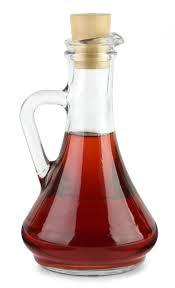Did you find an error or inaccuracy? Feel free to write us. Thank you!Tips to related online calculators
Looking for a statistical calculator?
Do you have a linear equation or system of equations and looking for its solution? Or do you have a quadratic equation?

## Related math problems and questions:

• Vinegar 2How many percentages of vinegar solution will we get if we mix 3.5 liters of 5.8% and 5 liters of 7.6% vinegar?
• Vinegar mixing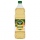We mix 70g water and 50g 8% vinegar. How many percents of the solution of vinegar we produce?
• PeroxideHow many ml should we pour 30% of peroxide (H2O2) into 100ml H2O to give a 20% solution?
• PharmacyThe pharmacy adds to 3 liters of 95-percent alcohol 5 liters of 38.04 percent alcohol. How much percent alcohol the pharmacy got?
• PharmacistThe pharmacist had a 12 l vessel herbal lotion, which contained a 30% active ingredient. Her assistant poured 18 liters of herbal lotion containing 45% of the active ingredient into a container. What percentage of herbal lotion was produced?
• Concentration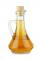How 0.5 liters of 8% vinegar diluted to a concentration of 20 hundredths % of vinegar? How many liters of water must be poured?
• Vinegar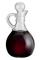We must dilute 16 liters of 8.8% aqueous vinegar to 4.1% one. How much water is necessary to add?
• Mixture 2How many liters of water must be added to 7 liters of a 20% solution to obtain a 10% solution?
• CuSO4 mixtureHow many grams of solid CuSO4 we have to add to 450g of 15% CuSO4 solution to produce a 25% solution?
• A 36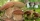A 36 g sample of the substance contains 91% water. After drying, the weight of the sample was reduced to 18 g. What percentage of water is now in the sample?
• Hydrochloric acidDetermine the concentration of which must have a solution of hydrochloric acid that mixing 10 l of the solution with 8 liters of 26% solution to get the solution with a concentration of 50%?
• Solutions, mixturesHow many liters of 70% solution must we add to 5 liters of 30% solution to give us a 60% solution?
• SolutionWhat amount of 80% solution and what amount of water is needed to mix in order to obtain two liters of 60% solution of this mixture?
• Final examThere are 5 learners in a class that has written a final exam Aleta scored 55% vera scored 36% and Sibusiso scored 88% if Thoko scored 71% and the class average was 63%. What was Davids score as a percentage?
• Sea waterMixing 62 kg of sea water with 84 kg rainwater is created water containing 3.1% salt. How many percent sea water contains salt?
• Sea waterSeawater contains about 4.3% salt. How many dm3 of distilled water we must pour into 5 dm3 of sea water to get water with 1.8% salt?
• Average mark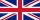Calculate average mark in English, if a student got a couple of 3's, 20% less 2 than 3, and 50% more 1's than the 2's.